• 偶然发现了一个神器，用来做前期的数据处理确实很方便。 做数据分析前需要先看一下数据的概况，有一个很好用的工具pandas_profiling可以快速预览数据 只用一行代码，即可快速显示数据概况 用 pip install pandas_...
偶然发现了一个神器，用来做前期的数据处理确实很方便。

做数据分析前需要先看一下数据的概况，有一个很好用的工具pandas_profiling可以快速预览数据

只用一行代码，即可快速显示数据概况

用 pip install pandas_profiling 安装就行。

以下是代码：

import pandas as pd
import pandas_profiling

df = pd.read_csv('shijingshan_air_outdoors(0606_0613).csv')     # 在这里输入想要分析的数据.csv文件

# windows pycharm执行代码,执行完在浏览器打开example.html
if __name__ == '__main__':
pfr = pandas_profiling.ProfileReport(df)
pfr.to_file("./example.html")



分析的结果会保存为一个HTML文件，用浏览器打开即可。

参考文档：

https://blog.csdn.net/adam_wzs/article/details/79245361

https://blog.csdn.net/Andy_shenzl/article/details/81709409

展开全文Python
• 如何计算两只股票方差相关性 考虑一个由“沃尔玛”和“Facebook”组成的投资组合。你认为这些公司的收益会显示出高或低的协方差吗？或者，你能猜出相关性是什么吗？它是接近0还是接近1？ 从2015年1月1日到今天，为...
如何计算两只股票方差和相关性
考虑一个由“沃尔玛”和“Facebook”组成的投资组合。你认为这些公司的收益会显示出高或低的协方差吗？或者，你能猜出相关性是什么吗？它是接近0还是接近1？
从2015年1月1日到今天，为沃尔玛和Facebook提取数据。
import numpy as np
import pandas as pd
from pandas_datareader import data as wb

# 沃尔玛  Fackbook
tickers = ['WMT', 'FB']
sec_data = pd.DataFrame()
for t in tickers:
sec_data[t] = wb.DataReader(t, data_source='iex', start='2015-1-1')['close']

sec_data.head()


WMT
FB

date

2015-01-02
76.1120
78.450

2015-01-05
75.8905
77.190

2015-01-06
76.4752
76.150

2015-01-07
78.5043
76.150

2015-01-08
80.1612
78.175

# 对数收益率
returns = np.log(sec_data / sec_data.shift(1))
returns.head()


WMT
FB

date

2015-01-02
NaN
NaN

2015-01-05
-0.002914
-0.016192

2015-01-06
0.007675
-0.013565

2015-01-07
0.026187
0.000000

2015-01-08
0.020886
0.026245

对这两支股票,如何解释他们的平均值和标准差之间的差异？
# 以一年为单位  平均值
returns[['WMT', 'FB']].mean() * 250

WMT    0.063984
FB     0.195344
dtype: float64

# 标准差
returns[['WMT', 'FB']].std() * 250 ** 0.5

WMT    3.184389
FB     4.536966
dtype: float64

# 方差
returns[['WMT', 'FB']].var() * 250

WMT    0.040561
FB     0.082336
dtype: float64

Covariance and Correlation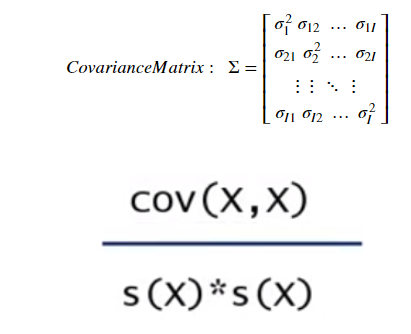# 天
cov_matrix = returns.cov()
cov_matrix


WMT
FB

WMT
0.000162
0.000034

FB
0.000034
0.000329

# 年
cov_matrix_a = returns.cov() * 250
cov_matrix_a


WMT
FB

WMT
0.040561
0.008430

FB
0.008430
0.082336

# 相关性
corr_matrix = returns.corr()
corr_matrix


WMT
FB

WMT
1.000000
0.145868

FB
0.145868
1.000000

沃尔玛和Facebook两只股票的协方差是0.145868，具有弱相关系
但是这不是两种股票价格的相关系，是回报的相关系


展开全文• [概统]本科二年级 概率论与数理统计 第七讲 期望、方差相关性
[概统]本科二年级 概率论与数理统计 第七讲 期望、方差与相关性期望及其性质方差、协方差、相关性系数两两独立、独立与相关性
虽然之前就分别介绍过离散型随机变量与连续型随机变量的期望与方差，这一讲我们正式介绍期望、方差的概念与性质。
期望及其性质
定义7.1

假设$X$是一个一元离散型随机变量，$\rho_X$是它的分布列，则它的期望是$E[X]=\sum x\rho_X(x)$
假设$X$是一个一元连续型随机变量，$f_X$是它的概率密度，则它的期望是$E[X]=\int xf_X(x)dx$
假设$X$是一个一元离散型随机变量，$\rho_{X|Y}(x|y)$是它的条件分布列，则它的条件期望是$E[X|Y=y]=\sum x\rho_{X|Y}(x|y)$
假设$X$是一个一元连续型随机变量，$f_{X|Y}(x|y)$是它的概率密度，则它的条件期望是$E[X|Y=y]=\int xf_{X|Y}(x|y)dx$

定义7.2 期望的存在性
如果$E[X]<\infty$，则称随机变量的期望存在。
定义7.3 随机变量的函数的期望
考虑函数$Z = g(X,Y)$，我们可以定义$Z$的期望为
$E[Z]=\iint g(x,y)f(x,y)dxdy$
如果$E[Z]<\infty$，则称$Z$的期望存在。
例7.1 Cauchy分布
上一讲例7我们基于正态分布导出了Cauchy分布，现在我们讨论标准Cauchy分布的期望的存在性，考虑$X \sim f_X$
$f_X(x) = \frac{1}{\pi} \frac{1}{1+x^2},x \in \mathbb{R}$
我们计算一下它的期望：
$\int_{-\infty}^{+\infty} \frac{x}{\pi} \frac{1}{1+x^2}dx = \lim_{y \to \infty}\int_{-\infty}^{y} \frac{x}{\pi} \frac{1}{1+x^2}dx \\ = \lim_{y \to \infty} \frac{\ln (y^2+1)}{2\pi} \to +\infty$
也就是说这个期望会发散，于是标准Cauchy分布没有期望，事实上任意Cauchy分布都是没有期望的。
定理7.1 期望与条件期望的关系
假设$X$的期望存在，则

如果$X$是离散型随机变量，则$E[X]=\sum E[X|Y=y]\rho_Y(y)$
如果$X$是连续型随机变量，则$E[X]=\int E[X|Y=y]f_Y(y)dy$

证明
第一条就是第五讲的定理5.4，我们证明一下第二条。
因为$E[X]<\infty$，我们可以使用Fubini定理，计算
$E[X] = \int xf_X(x)dx=\int x \int f(x,y)dydx \\ = \iint xf(x,y)dxdy = \int dy \int xf(x,y)dx$
根据条件密度的定义，
$f(x,y) = f_{X|Y}(x|y)f_Y(y)$
所以
$\int dy \int xf(x,y)dx = \int dy \int xf_{X|Y}(x|y)f_Y(y)dx \\ = \int E[X|Y=y]f_Y(y)dy$
定理7.2 期望的性质

常数的期望是它本身: $\forall C \in \mathbb{R},E[C]=C$
期望的线性性：如果$X,Y$的期望存在，$\forall a,b \in \mathbb{R},E[aX+bY]=aE[X]+bE[Y]$
如果$X,Y$独立，并且$XY$的期望存在，则$E[XY]=E[X]E[Y]$

证明
第二条性质的证明留给读者，这里简单叙述一下第三条性质的证明：根据定义
$E[XY] = \iint xy f(x,y)dxdy$
因为$X,Y$独立，$f(x,y)=f_X(x)f_Y(y)$，根据Fubini定理，
$\iint xy f(x,y)dxdy = \iint xy f_X(x)f_Y(y)dxdy \\ = \int xf_X(x)dx \int yf_Y(y)dy = E[X]E[Y]$
例7.2 计算E[F(X)]
假设$X$是一个一元连续型随机变量，累积分布函数为$F$，求$E[F(X)]$。
这是一个比较有趣的题目，$E$代表期望、$F$代表累积分布、$X$代表任意连续型随机变量，然而把这三个东西合在一起却能得到一个常数：
记$Y=F(X)$，根据累积分布函数的性质$Y \in [0,1]$，$\forall y \in [0,1]$，
$P(Y \le y)=P(F(X) \le y) = P(X \le F^{-1}(y)) \\ =F(F^{-1}(y))=y$
也就是说$Y$服从$[0,1]$上的均匀分布，所以
$E[F(X)]=E[Y]=1/2$
例7.3 虫族的故事续
第五讲例5.6我们分析了高级虫族的数量，现在我们分析一下虫族的能源：
某虫族在任意时刻开采的辉晶矿含量为$X$，$X$服从参数为$\lambda$的指数分布，这些辉晶矿通过合光阵可以产出$Y$千焦机械能，其中$Y$服从正态分布$N(\mu X,\sigma^2)$

求$(X,Y)$的联合分布；
求$E[Y]$

第一问，根据题目的含义，$Y|X=x \sim N(\mu x,\sigma^2)$，
$f_{Y|X}(y|x) = \frac{1}{\sqrt{2\pi} \sigma}e^{-\frac{(y-\mu x)^2}{2\sigma^2}}$
因为$X$服从参数为$\lambda$的指数分布，
$f_X(x) = \lambda e^{-\lambda x},x \ge 0$
所以根据定义
$f(x,y) =f_{Y|X}(y|x)f_X(x)= \frac{\lambda}{\sqrt{2\pi} \sigma}e^{-\lambda x-\frac{(y-\mu x)^2}{2\sigma^2}},x \ge 0$
第二问，先计算条件期望
$E[Y|X=x]=\mu x$
根据定理7.1，
$E[Y]=\int_{0}^{\infty} \mu x \lambda e^{-\lambda x}=\frac{\mu}{\lambda}$
方差、协方差、相关性系数
定义7.4 方差与二阶原点矩
假设$X$的期望存在，记$Var(X)$为$X$的方差，如果
$Var(X) = E[(X-E[X])^2]$
如果$Var(X)<\infty$，称$X$的方差存在；称$E[X^2]$为$X$的二阶原点矩，另外$Var(X)$又叫做二阶中心矩。称$sd(X)$为$X$的标准差，简记为$\sigma_X$，如果
$sd(X) = \sqrt{Var(X)}$
评注
$Var(X)=E[X^2-2XE[X]+(E[X])^2] \\=E[X^2]-2(E[X])^2+(E[X])^2 = E[X^2]-(E[X])^2$
定理7.3 方差的性质

$Var(X+c)=Var(X)$
$Var(aX)=a^2Var(X)$
如果$X,Y$独立，则$Var(X+Y)=Var(X)+Var(Y)$

证明
第一个性质
$E[(X+c)^2]=E[X^2+2cX+c^2]=E[X^2]+2cE[X]+c^2 \\ (E[X+c])^2=(E[X]+c)^2=(E[X])^2+2cE[X]+c^2 \\ Var(X+c)=E[X^2]-(E[X])^2=Var(X)$
第二个性质
$E[(aX)^2]=E[a^2X^2]=a^2E[X^2] \\ (E[aX])^2 = a^2(E[X])^2 \\ Var(aX)=a^2E[X^2]-a^2(E[X])^2 =a^2Var(X)$
第三个性质，
$Var(X+Y)=E[(X+Y)^2]-(E[X+Y])^2$
其中第二项为$(E[X+Y])^2 = (E[X]+E[Y])^2 \\=(E[X])^2+2E[X]E[Y]+(E[Y])^2$
第一项为
$E[(X+Y)^2]=E[X^2+2XY+Y^2] \\ =E[X^2]+2E[XY]+E[Y^2]$
因为$X,Y$独立，$E[XY]=E[X]E[Y]$，于是
$Var(X+Y)=E[X^2]-(E[X])^2+E[Y^2]-(E[Y])^2 \\ =Var(X+Y)$
定义7.5 协方差与相关性系数
考虑两个随机变量$X,Y$，记$Cov(X,Y)$为$X,Y$的协方差，简记为$\sigma_{XY}$，如果
$Cov(X,Y)=E[(X-E[X])(Y-E[Y])]$
记$\rho(X,Y)$表示$X,Y$的相关性系数，如果
$\rho(X,Y) = \frac{Cov(X,Y)}{\sqrt{Var(X)Var(Y)}}=\frac{Cov(X,Y)}{sd(X)sd(Y)}$
如果$\rho(X,Y)>0$，称$X,Y$正相关；如果$\rho(X,Y)<0$，称$X,Y$负相关；如果$\rho(X,Y)=0$，称$X,Y$不相关或$X,Y$正交，因为相关性系数的分母为正，所以相关性系数的符号等于协方差的符号。
如果$X=(X_1,X_2)$是一个二元随机变量，称$Cov(X)$是$X$的协方差矩阵，
$Cov(X)=E[(X-E[X])(X-E[X])^T]$
其中
$E[X] =\left[\begin{matrix} E[X_1] \\ E[X_2] \end{matrix}\right]$
所以
$Cov(X) = \left[\begin{matrix} \sigma_{X_1}^2 & \sigma_{XY} \\ \sigma_{XY} & \sigma^2_{X_2} \end{matrix}\right]$
评注
$Cov(X,Y)=E[XY-E[X]Y-XE[Y]+E[X]E[Y]] \\ = E[XY]-E[X]E[Y]$
定理7.4 协方差与相关性系数的性质

$Cov(X,Y)=Cov(Y,X)$
$Cov(aX,bY)=abCov(X,Y)$
$Cov(X+Y,Z)=Cov(X,Z)+Cov(Y,Z)$
$\rho(X,Y) \in [-1,1]$，等于$\pm 1$的充要条件是$Y=a+bY,\exists a,b \in \mathbb{R}$

这四条性质留给读者证明，可以参考盛骤的第四章第三节。
例7.4 二元正态分布
假设$(X_1,X_2)$服从标准二元正态分布（参考第五讲），我们可以根据$(X_1,X_2)$构造相关性系数为$\rho$的正态分布，定义
$Z_1 = X_1,\ \ Z_2 = \rho X_1 + \sqrt{1-\rho^2}X_2$
根据随机变量变换的规则（参考第六讲微分同胚的分布），
$f_{Z_1,Z_2}(z_1,z_2) = \frac{1}{2\pi\sqrt{1-\rho^2}} \exp \left( -\frac{z_1^2 + (\frac{z_2-\rho z_1}{\sqrt{1-\rho^2}})^2}{2} \right) \\ = \frac{1}{2\pi\sqrt{1-\rho^2}} \exp \left( -\frac{z_1^2 -2\rho z_1z_2 + z_2^2}{2(1-\rho^2)} \right)$
我们可以发现
$f_{Z_1}(z_1) = \int_{-\infty}^{+\infty} \frac{1}{2\pi\sqrt{1-\rho^2}} \exp \left( -\frac{z_1^2 -2\rho z_1z_2 + z_2^2}{2(1-\rho^2)} \right) dz_2 \\ = \int_{-\infty}^{+\infty} \frac{e^{-\frac{z_1^2}{2}}}{2\pi\sqrt{1-\rho^2}}\exp \left( -\frac{(z_2-\rho z_1)^2}{2(1-\rho^2)} \right) dz_2 \\ = \frac{\sqrt{2\pi (1-\rho^2)}e^{-\frac{z_1^2}{2}}}{2\pi\sqrt{1-\rho^2}} = \frac{1}{\sqrt{2\pi}} e^{-\frac{z_1^2}{2}}$
也就是说$Z_1$和$Z_2$的边缘密度还是标准正态的。
例7.5 资本资产定价模型CAPM
考虑一元线性回归$Y=\beta_0+\beta_1 X+u,u\sim N(0,\sigma^2)$，$u$与$X$不相关，假设$X$的期望与方差存在，求$\beta_1$，并分析$\beta_1$与相关性系数的关系。在资本资产定价模型中，$Y$表示某项资产的回报率，$X$表示市场的风险溢价(市场回报率减去无风险利率)，$\beta_1$表示资产的beta，这是对资产包含的系统性风险的度量，beta越大，说明资产包含的系统性风险越多，回报率也就越高，$\beta_0$表示资产的alpha，它表示当市场不均衡时，资产可能出现的超额回报，基于alpha与beta可以构造用于套利的alpha策略，$u$表示资产的个别风险，在CAPM的观点中，个别风险不会带来回报。
因为$Cov(X,u)=0$，既然涉及到相关性系数了，那么我们先计算一下协方差,
$Cov(X,Y)=Cov(X,\beta_0+\beta_1 X+u) \\=Cov(X,\beta_0)+\beta_1Cov(X,X)+Cov(X,u)$
其中第三项为0，因为$\beta_1,\beta_0$是常数，所以第一项为
$Cov(X,\beta_0)=E[X\beta_0]-\beta_0E[X]=0$
第二项为
$Cov(X,X)=E[X^2]-(E[X])^2=Var(X)$
于是
$Cov(X,Y) =\beta_1Var(X)\Rightarrow \beta_1 = \frac{Cov(X,Y)}{Var(X)}$
相关性系数的定义是
$\rho_{XY} = \frac{Cov(X,Y)}{\sqrt{Var(X)Var(Y)}} \Rightarrow Cov(X,Y)=\rho_{XY}\sqrt{Var(X)Var(Y)}$
因此
$\frac{Cov(X,Y)}{Var(X)} = \frac{\rho_{XY}\sqrt{Var(X)Var(Y)}}{Var(X)}=\rho_{XY}\sqrt{\frac{Var(Y)}{Var(X)}}$
也就是说
$\beta_1=\rho_{XY}\sqrt{\frac{Var(Y)}{Var(X)}}$
例7.6 虫族的故事续

某虫族在任意时刻有母体数目$X$个，$X$服从参数为$\lambda$的Poisson分布，这些母体可以产出$Y$个高级虫族，其中$Y$服从参数为$X+1$的指数分布，计算$Cov(X,Y)$
某虫族在任意时刻开采的辉晶矿含量为$X$，$X$服从参数为$\lambda$的指数分布，这些辉晶矿通过合光阵可以产出$Y$千焦机械能，其中$Y$服从正态分布$N(\mu X,\sigma^2)$，计算$Cov(X,Y)$

第一问，在例5.6中我们得到了$X,Y$的联合分布与$E[Y]$，
$f(x,y) = \frac{(x+1)\lambda^xe^{-(\lambda+(x+1)y)}}{x!},y \ge 0,x=0,1,\cdots \\ E[Y]=\frac{1-e^{-\lambda}}{\lambda}$
因为$X$服从参数为$\lambda$的Poisson分布，$E[X]=\lambda$，要计算$Cov(X,Y)$，我们只需要计算$E[XY]$即可，
$\int_0^{+\infty}\sum_{x=0}^{+\infty} xy\frac{(x+1)\lambda^xe^{-(\lambda+(x+1)y)}}{x!}dy$
这个式子乍一看是有点复杂的，但在积分与级数都收敛的时候，他们可以交换次序，因此我们可以先计算更简单的积分的部分
$\sum_{x=0}^{+\infty} \frac{x\lambda^x}{x!}\int_0^{+\infty}(x+1)e^{-(\lambda+(x+1)y)}dy \\ = \sum_{x=0}^{+\infty} \frac{x\lambda^xe^{-\lambda}}{x!(x+1)}= \sum_{x=0}^{+\infty} \frac{\lambda^xe^{-\lambda}}{x!}- \sum_{x=0}^{+\infty} \frac{\lambda^xe^{-\lambda}}{(x+1)!}$
最后一步是对分子上的$x$做拆分，$x = (x+1)-1$，第一项就是Poisson分布的归一性条件，因此第一项为1，接下来讨论第二项，
$\sum_{x=0}^{+\infty} \frac{\lambda^xe^{-\lambda}}{(x+1)!}=\frac{1}{\lambda}\sum_{x=0}^{+\infty} \frac{\lambda^{x+1}e^{-\lambda}}{(x+1)!} = \frac{1}{\lambda}\sum_{x=1}^{+\infty} \frac{\lambda^{x}e^{-\lambda}}{x!} = \frac{1}{\lambda}(1-e^{-\lambda})$
所以
$E[XY]=1-\frac{1}{\lambda}(1-e^{-\lambda})$
于是
$Cov(X,Y)=E[XY]-E[X]E[Y] \\=1-\frac{1}{\lambda}(1-e^{-\lambda})-\lambda\frac{1}{\lambda}(1-e^{-\lambda}) \\ = 1-\frac{1}{\lambda}(1-e^{-\lambda})-1+e^{-\lambda}=e^{-\lambda}-\frac{1}{\lambda}(1-e^{-\lambda})$
第二问，在例7.3中我们得到了联合分布与$E[Y]$，
$f(x,y) =f_{Y|X}(y|x)f_X(x)= \frac{\lambda}{\sqrt{2\pi} \sigma}e^{-\lambda x-\frac{(y-\mu x)^2}{2\sigma^2}},x \ge 0 \\ E[Y] = \mu/\lambda$
因为$X$服从参数为$\lambda$的指数分布，$E[X]=1/\lambda$，于是我们只需要计算$E[XY]$，
$E[XY]=\int_{-\infty}^{+\infty}\int_0^{+\infty}\frac{\lambda xy}{\sqrt{2\pi} \sigma}e^{-\lambda x-\frac{(y-\mu x)^2}{2\sigma^2}}dxdy$
我们简单观察一下这个积分就会发现，先积$x$并不明智，因为关于$x$的表达式太复杂了，所以这个积分存在时，我们最好交换一下积分次序，先积$y$，
$\int_0^{+\infty}\lambda xe^{-\lambda x}\int_{-\infty}^{+\infty}y\frac{1}{\sqrt{2\pi} \sigma}e^{-\frac{(y-\mu x)^2}{2\sigma^2}}dydx$
注意到
$\int_{-\infty}^{+\infty}y\frac{1}{\sqrt{2\pi} \sigma}e^{-\frac{(y-\mu x)^2}{2\sigma^2}}dy=E[Y|X=x]=\mu x$
于是我们再对$x$积分
$\int_0^{+\infty}\lambda \mu x^2e^{-\lambda x}dx = \mu E[X^2]=\mu(Var(X)+(E[X])^2) = \frac{2\mu}{\lambda^2}$
这样我们就可以计算协方差了
$Cov(X,Y)=E[XY]-E[X]E[Y]=\frac{2\mu}{\lambda^2}-\frac{\mu}{\lambda^2}=\frac{\mu}{\lambda^2}$
例7.8 Markowitz投资组合理论
假设资产$A$的收益率均值为$\mu_A$，波动率(即标准差)为$\sigma_A$，资产$B$的收益率均值为$\mu_B$，波动率为$\sigma_B$，二者收益率相关性系数为$\rho$，是否存在用资产$A$与资产$B$构造的投资组合，使得组合收益率等于$r$时风险最小？
假设这个资产组合中$A$的权重为$w$，则$B$的权重为$1-w$，于是资产组合收益率均值为$w\mu_A+(1-w)\mu_B$，记$X$表示资产$A$的收益率，$Y$表示资产$B$的收益率，则资产组合收益率的方差为
$Var(wX+(1-w)Y) \\=E[(wX+(1-w)Y)^2]-(E[wX+(1-w)Y])^2$
第一项为
$E[(wX+(1-w)Y)^2]=E[w^2X^2+2w(1-w)XY+(1-w)^2Y^2] \\ = w^2E[X^2]+2w(1-w)E[XY]+(1-w)^2E[Y^2]$
第二项为
$(E[wX+(1-w)Y])^2=(wE[X]+(1-w)E[Y])^2 \\ = w^2(E[X])^2+2w(1-w)E[X]E[Y]+(1-w)^2(E[Y])^2$
所以
$Var(wX+(1-w)Y) \\=w^2Var(X)+2w(1-w)Cov(X,Y)+(1-w)^2Var(Y)$
也就是说投资组合收益率的方差为
$w^2\sigma_A^2+2w(1-w)\rho \sigma_A\sigma_B+(1-w)^2\sigma_B^2$
也就是说我们要做优化：
$\min_{w}w^2\sigma_A^2+2w(1-w)\rho \sigma_A\sigma_B+(1-w)^2\sigma_B^2 \\ w\mu_A+(1-w)\mu_B = r$
剩下的留给读者完成，这里关于两个随机变量和的方差的公式是具有一般性的。
两两独立、独立与相关性
定义7.6 独立性

正交：$Cov(X,Y)=0$，则$X,Y$正交；
两两独立：$X,Y,Z$中任两个独立，比如$f(x,y)=f_X(x)f_Y(y)$，则它们两两独立；
独立：$X,Y,Z$独立，则$X,Y,Z$两两独立，并且$f(x,y,z)=f_X(x)f_Y(y)f_X(x)$

说明
这四条是关于独立性与相关性的定义，其中独立最强，两两独立次之，正交最弱。我们用下面两个例子说明它们的强弱关系。
例7.9 正交但不独立
假设$(X,Y)$服从$\{(x,y):x^2+y^2=1\}$上的均匀分布，也就是类似我们在第二讲讨论Bertrand悖论时一样，假设点在圆周上是均匀的。下面我们说明两点：

$X,Y$正交
$X,Y$不独立

第一问，因为$\{(x,y):x^2+y^2=1\}$的圆心在原点，所以$EX=EY=0$（因为关于原点对称）。根据这种中心对称性，我们还可以得到一个结论：
$E(-XY)=E(XY) \Rightarrow E(XY)=0$
于是$Cov(X,Y)=0$；
第二问，举出一个反例即可
$P(X_1>a)P(X_2>a) \ne 0,\forall a \in (1/\sqrt{2},1) \\ P(X_1>a,X_2>a)=0$
即$X_1,X_2$不独立。
例7.10 两两独立但不独立
假设$X,Y,Z$的概率密度如下：
$f(x,y,z) = \begin{cases} \frac{1-\sin x \sin y \sin z}{8 \pi^3},0 \le x,y,z \le 2\pi \\ 0 ,otherwise \end{cases}$
$X,Y,Z$两两独立但不独立，读者可以自行验证这个结论。


展开全文• －在我们的工作中，会有一个这样的场景，有若干数据罗列在我们的面前，这组数据相互之间可能会存在一些联系，可能是此增彼涨，或者是负相关，也可能是没有关联，那么...关键词python方差协方差相关系数离散度pand...


－　点击上方“中国统计网”订阅我吧！－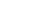在我们的工作中，会有一个这样的场景，有若干数据罗列在我们的面前，这组数据相互之间可能会存在一些联系，可能是此增彼涨，或者是负相关，也可能是没有关联，那么我们就需要一种能把这种关联性定量的工具来对数据进行分析，从而给我们的决策提供支持，本文即介绍如何使用 Python 进行数据相关性分析。 关键词 python 方差 协方差 相关系数 离散度 pandas numpy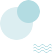实验数据准备接下来，我们将使用 Anaconda 的 ipython 来演示如何使用 Python 数据相关性分析，我所使用的 Python 版本为 3.6.2 。首先，我们将会创建两个数组，数组内含有 20 个数据，均为 [0, 100] 区间内随机生成。a = [random.randint(0, 100) for a in range(20)]b = [random.randint(0, 100) for a in range(20)]print(a)>> [35, 2, 75, 72, 55, 77, 69, 83, 3, 46, 31, 91, 72, 12, 15, 20, 39, 18, 57, 49]print(b)>> [25, 24, 72, 91, 27, 44, 85, 21, 0, 64, 44, 31, 6, 91, 1, 61, 5, 39, 24, 43期望在进行相关性分析之前，我们需要先为最终的计算分析做好准备。我们在分析前，第一个准备的是计算数据的期望。对于期望的定义，离散变量和连续变量是不一样的，具体定义如下： - 对于连续随机变量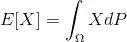在离散随机变量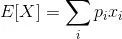在一般情况下，我们通过实验或者调查统计获取的数据很大一部分都属于离散随机变量，那么这里的期望我们也可以简单的理解为平均数，那么既然是平均数，那么我们就可以非常简单编写一个计算离散变量的期望的函数了。def mean(x):  return sum(x) / len(x)mean(a)>> 46.05mean(b)>> 39.9离散度 - 方差与标准差接下来，我们需要计算的是数据的离散程度，在统计上，我们通常会使用方差和标准差来描述。 方差和期望一样，对于连续和离散的随机变量有着不同的定义，具体定义如下： 对于连续随机变量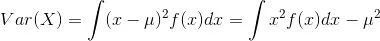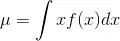对于离散随机变量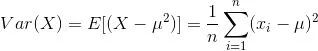与期望类似，这里我们一般只考虑离散变量的方差。还有一点值得注意，我们上面的离散变量方差公式，最后是除以 n，但实际上，我们计算样本方差的时候一般会使用 n-1。而标准差，就是方差的平方根。那么，我们也可以像上面计算期望一样，给方差和标准差编写函数。# 计算每一项数据与均值的差def de_mean(x):  x_bar = mean(x)  return [x_i - x_bar for x_i in x]# 辅助计算函数 dot product 、sum_of_squaresdef dot(v, w):  return sum(v_i * w_i for v_i, w_i in zip(v, w))def sum_of_squares(v):  return dot(v, v)# 方差def variance(x):  n = len(x)  deviations = de_mean(x)  return sum_of_squares(deviations) / (n - 1)# 标准差import mathdef standard_deviation(x):  return math.sqrt(variance(x))variance(a)>> 791.8394736842105varance(b)>> 850.5157894736841协方差与相关系数接下来，我们进入正题，我们开始计算两组数据的相关性。我们一般采用相关系数来描述两组数据的相关性，而相关系数则是由协方差除以两个变量的标准差而得，相关系数的取值会在 [-1, 1] 之间，-1 表示完全负相关，1 表示完全相关。接下来，我们看一下协方差和相关系数的定义： 协方差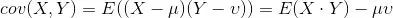相关系数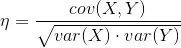同样的，我们根据上述的公式编写函数。# 协方差def covariance(x, y):  n = len(x)  return dot(de_mean(x), de_mean(y)) / (n -1)# 相关系数def correlation(x, y):  stdev_x = standard_deviation(x)  stdev_y = standard_deviation(y)  if stdev_x > 0 and stdev_y > 0:    return covariance(x, y) / stdev_x / stdev_y  else:    return 0covariance(a, b)>> 150.95263157894735correlation(a, b)>> 0.18394200852440826根据上面的结果，相关系数为 0.18，可以推断这两组随机数有弱正相关。当然，我们知道，这两组数据都是使用 random 函数随机生成出来的，其实并没有什么相关性，这也是在数据处理中，需要特别留意的一个地方，统计的方法可以给我们一个定量的数值可供分析，但实际的分析也需要结合实际以及更多的情况综合考虑。使用 numpy 计算协方差矩阵 相关系数一般我们日常工作，都不会像上面一样把什么期望、方差、协方差一类的函数都重新写一遍，上面的代码只是让我们对这些计算更加熟悉。我们通常情况下会使用 numpy 一类封装好的函数，以下将演示一下如何使用 numpy 计算协方差。import numpy as np# 先构造一个矩阵ab = np.array([a, b])# 计算协方差矩阵np.cov(ab)>> array([[ 791.83947368,  150.95263158],       [ 150.95263158,  850.51578947]])这里我们可以看到，这里使用 np.cov 函数，输出的结果是一个矩阵，这就是协方差矩阵。协方差矩阵数据的看法也不难，我们可以以上面的结果为例，矩阵1行1列，表示的是 a 数据的方差，这和我们上面的计算结果一致，然后1行2列和2行1列分别是 a b 以及 b a 的协方差，所以他们的值是一样的，然后最后2行2列就是 b 数据的方差。接下来，我们继续使用 numpy 计算相关系数np.corrcoef(ab)>> array([[ 1.        ,  0.18394201],       [ 0.18394201,  1.        ]])计算相关系数，我们使用 numpy 的 corrcoef 函数，这里的输出也是一个矩阵，这个矩阵数据的含义同上面的协方差类似，我们可以看到，这里我们的相关系数是 0.18 ，和我们上面自己编写的函数计算的结果一致。使用 pandas 计算协方差、相关系数除了使用 numpy，我们比较常用的 python 数据处理库还有 pandas，很多金融数据分析的框架都会使用 pandas 库，以下将演示如何使用 pandas 库计算协方差和相关系数。import pandas as pd# 使用 DataFrame 作为数据结构，为方便计算，我们会将 ab 矩阵转置dfab = pd.DataFrame(ab.T, columns=['A', 'B'])# A B 协方差dfab.A.cov(dfab.B)>> 150.95263157894738# A B 相关系数dfab.A.corr(dfab.B)>>  0.18394200852440828dfab>>    A   B0   35  251    2  242   75  723   72  914   55  275   77  446   69  857   83  218    3   09   46  6410  31  4411  91  3112  72   613  12  9114  15   115  20  6116  39   517  18  3918  57  2419  49  43可以看到，和 numpy 相比，pandas 对于有多组数据的协方差、相关系数的计算比 numpy 更为简便、清晰，我们可以指定计算具体的两组数据的协方差、相关系数，这样就不需要再分析结果的协方差矩阵了。小结本文通过创建两组随机的数组，然后通过参考定义公式编写函数，再到使用 numpy 以及 pandas 进行协方差、相关系数的计算。到这里我们应该已经了解了数据相关性分析的原理，以及简单的具体实践使用方法，日后在工作中遇到需要做数据相关性分析的时候，就可以派上用场了。End.作者：空空来源：知乎零基础入职数据分析就业班课程的形式主要是“直播+录播”报名专享：课程项目作业+1v1班主任监督学习+爱数据学院学员专属网站+班级答疑群课程结束后能熟练掌握SQL、Python、Excel、PPT等工具适合人群：1.转行(岗位相关，专业相关、对数据分析感兴趣)2.从事数据分析工作，但是需要提升技能以及增加实战经验3.应届毕业生入职数据分析展开全文• https://www.jianshu.com/p/1e5389ca9829多重共线性
• UA MATH571A 一元线性回归III 一元线性回归的ANOVAANOVA TableF检验回归系数的F...ANOVA（Analysis of Variance）是分析方差构成的常用方法。在前两篇中，我们定义过 SST=∑i=1N(Yi−Yˉ)2 SST = \sum_{i=1}^N (...
• 指标解释：所有样本的样本误差的绝对值占实际值的比值 指标解读：指标越接近与0，模型越准确 5)模型解释度：R squared R方 r2 指标解释：应变量的方差能被自变量解释的程度 指标解读：指标越接近1，则代表自变量对于...
• 在我们的工作中，会有一个这样的场景，有若干数据罗列在我们的面前，这组数据相互之间可能会存在一些联系，可能是此增彼涨，或者是负相关...关键词python方差协方差相关系数离散度pandasnumpy实验数据准备接下来，我...
• 在我今天参与的一个讨论中，提出了一个问题，即在具有单个连续预测器的线性回归模型中R平方如何/是否取决于预测变量的方差。这个问题的答案当然是肯定的。 可视化 我们还可以在R中轻松地可视化前面的概念。我们...
• ## Python 数据相关性分析

万次阅读 多人点赞 2018-04-25 15:09:49
概述 在我们的工作中，会有一个这样的场景，有若干数据罗列在我们的面前，这组数据相互之间可能会存在一些联系，可能是此增彼涨，或者...关键词 python 方差 协方差 相关系数 离散度 pandas numpy 实验数据准备 ...python pandas numpy
• 若只考虑事件来研究事件的性质(概率，期望，方差相关性，独立性……)，当事件足够多的情况下，研究无法进行。这就必须将事件量化，引进随机变量。当然随机变量也分离散和连续的，其中离散随机变量可以看成是退化...
• 在统计学中，单因素方差分析是(one-way analysis of variance，缩写one-way ANOVA)一种常用于比较多个样本均值的方法。在之前的《如何进行单因素方差分析？》介绍了如何用SPSS在绘制图表前进行方差分析，并在《显著...
• R语言详解参数检验和非参数检验二、参数检验R语言实现2.1 单样本t检验2.2 独立样本t检验2.3 配对样本t检验2.4方差分析2.5 pearson相关性检验三、非参数检验R语言实现3.1单样本wilcoxon检验3.2 Mann-Whitney检验3.3...数据分析 机器学习 数据挖掘
• 有的材料按照 一维离散 -> 一维连续 -> 多维离散 -> 多维连续的角度讲解。...Geometric 和 Binomial 分布的 PMF，期望，方差公式和推导过程。目录随机变量定义。是 function，而非 variableP......